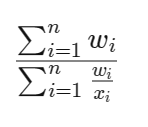# Harmonic Mean

## Harmonic Mean Definition

The harmonic mean is an average type useful for ratios or rates. It averages data by dividing the total number of values by the sum of each value's reciprocal. Unlike other averages, harmonic mean equally considers all data points, making it ideal for values in a ratio or rate relationship.

In finance, the harmonic mean assists in calculating averages for financial multiples like the price-to-earnings (P/E) ratio. It helps avoid bias towards larger values, which can distort the overall average when using the arithmetic mean. The harmonic mean offers a balanced approach by equally considering each value, making it suitable for different securities in a portfolio.

## Harmonic Mean Basics

The harmonic mean simplifies the relationship between fractions, useful for averaging rates like the average travel speed over multiple trips.

In finance, the weighted harmonic mean is beneficial for averaging multiples like the P/E ratio. This method ensures fair representation by assigning equal weight to each data point.

Using a weighted arithmetic mean would unfairly prioritize higher data points due to the lack of price normalization, while equalizing earnings.

The harmonic mean is a special type of weighted harmonic mean where each data point's weights are equal to 1. In a weighted harmonic mean, values are multiplied by their weights, summed, and then divided by the total weights sum. This can be mathematically represented as:The result is the weighted harmonic mean of x1, x2, x3, using weights w1, w2, w3.